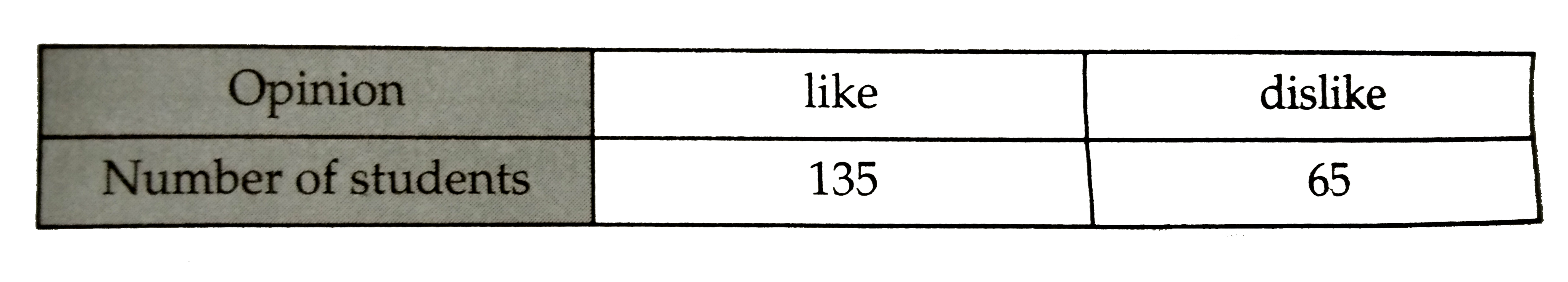# To know the opinion of the students about the subject Sanskrit , a survey of 200 students was obtained . The data is recorded as under . What is the p

35 views

closed
To know the opinion of the students about the subject Sanskrit , a survey of 200 students was obtained . The data is recorded as under .What is the probability that a student chosen at random does not like it ?
A. (13)/(27)
B. (27)/(40)
C. (13)/(40)
D. (27)/(13)

by (97.1k points)
selected by

Correct Answer - C
Total number of students = 200.
Number of students who do not like Sanskrit = 65 .
Required probability = (65)/(200) = (13)/(40) .